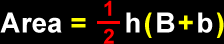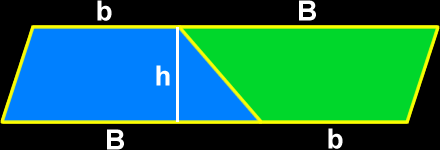# The Properties of a Trapezoid

Definitions and formulas for the perimeter of a trapezoid, the area of a trapezoid, properties of the sides and angles of a trapezoid, properties of the sides and angles of an isosceles trapezoid
Just scroll down or click on what you want and I'll scroll down for you!

 perimeter of a trapezoid area of a trapezoid sides and anglesof a trapezoid properties of theisosceles trapezoid

 The perimeter of a trapezoid:To find the perimeter of a trapezoid, just add up all the lengths of the sides:Perimeter = a + b + c + BThe area of a trapezoid:To find the area of a trapezoid...  The longer base (the bottom) is big B and the smaller base (the top) is little b...Yeah, that's the formula...  But let's check out where it comes from!
 Take two copies of the trapezoid (one blue trapezoid and one green trapezoid)... Tip one upside down and stick them together...  Now, you've got a parallelogram.  Area of a parallelogram = base x height                                     = ( B + b ) x h But, this is double of what we need...  So, multiply by 1/2!